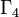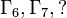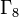Linear representation theory of groups of order 64

This article gives specific information, namely, linear representation theory, about a family of groups, namely: groups of order 64.
View linear representation theory of group families | View linear representation theory of groups of a particular order |View other specific information about groups of order 64
To understand these in a broader context, see: linear representation theory of groups of order 2^n|linear representation theory of groups of prime-sixth order

Degrees of irreducible representations

FACTS TO CHECK AGAINST FOR DEGREES OF IRREDUCIBLE REPRESENTATIONS OVER SPLITTING FIELD:
Divisibility facts: degree of irreducible representation divides group order | degree of irreducible representation divides index of abelian normal subgroup
Size bounds: order of inner automorphism group bounds square of degree of irreducible representation| degree of irreducible representation is bounded by index of abelian subgroup| maximum degree of irreducible representation of group is less than or equal to product of maximum degree of irreducible representation of subgroup and index of subgroup
Cumulative facts: sum of squares of degrees of irreducible representations equals order of group | number of irreducible representations equals number of conjugacy classes | number of one-dimensional representations equals order of abelianization

Grouping by degrees of irreducible representations

Note that since number of conjugacy classes in group of prime power order is congruent to order of group modulo prime-square minus one, all the values for the total number of irreducible representations, which equals the number of conjugacy classes, is congruent to 64 mod 3, and hence congruent to 1 mod 3.

Number of irreps of degree 1 Number of irreps of degree 2 Number of irreps of degree 4 Total number of irreps (= number of conjugacy classes) Total number of groups Nilpotency class(es) attained by these Hall-Senior family/families List of GAP IDs (second part)
64 0 0 64 11 1$\Gamma_1$, all the abelian groups of order 64 [SHOW MORE]
32 8 0 40 31 2$\Gamma_2$ [SHOW MORE]
32 0 2 34 7 2$\Gamma_5$ [SHOW MORE]
16 12 0 28 60 2,3$\Gamma_3$ (class three),$\Gamma_4$ (class two) [SHOW MORE]
16 4 2 22 41 2,3$\Gamma_6, \Gamma_7, ?$ [SHOW MORE]
8 14 0 22 33 2,3,4  ?,$\Gamma_8$ [SHOW MORE]